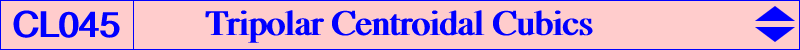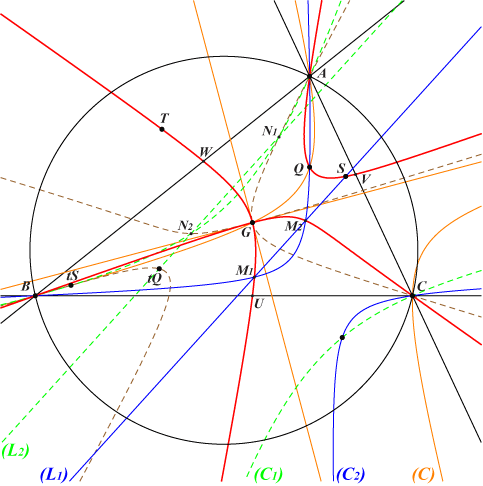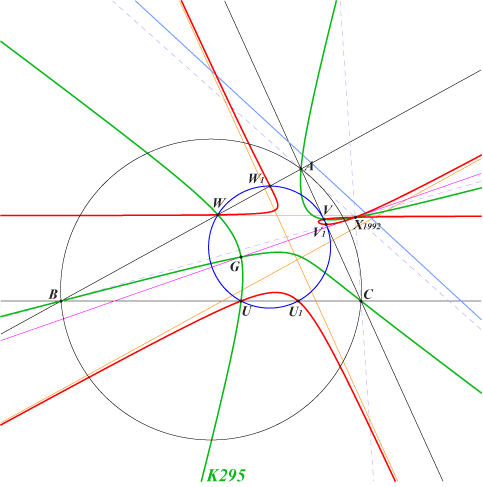The Tripolar Centroid TG(M) of a point M (distinct of G) is the isobarycenter of the traces of the trilinear polar of M on the sidelines of ABC. This notion was introduced by Darij Grinberg and Wilson Stothers has written an excellent note on this subject available here. TG(M) is also the intersection of the trilinear polar L of M and the trilinear polar of the infinite point of L. The list (by Peter Moses) gives a selection of pairs {M, TG(M)} : {1,1635}, {3,1636}, {4,1637}, {5,14391}, {6,351}, {7,1638}, {8,1639}, {9,14392}, {10,4120}, {11,14393}, {12,14394}, {13,9200}, {14,9201}, {17,14446}, {18,14447}, {21,14395}, {22,14396}, {24,14397}, {25,14398}, {27,11125}, {28,14399}, {29,14400}, {30,14401}, {31,14402}, {32,14403}, {37,14404}, {38,14405}, {39,14406}, {42,14407}, {43,14408}, {44,14409}, {45,14410}, {55,14411}, {56,14412}, {57,14413}, {63,14414}, {65,14415}, {66,14417}, {69,14417}, {75,4728}, {76,9148}, {78,14418}, {81,14419}, {83,14420}, {86,4750}, {88,14421}, {89,14422}, {98,1640}, {99,1641}, {100,1642}, {105,1643}, {111,9171}, {115,14423}, {141,14424}, {145,14425}, {190,1644}, {192,14426}, {200,14427}, {239,4448}, {251,14428}, {262,3569}, {263,2491}, {298,9204}, {299,9205}, {306,14429}, {312,14430}, {321,14431}, {333,14432}, {350,14433}, {385,11183}, {512,1645}, {513,1646}, {514,1647}, {519,6544}, {523,1648}, {524,1649}, {525,1650}, {536,14434}, {551,14435}, {648,1651}, {671,8371}, {869,14436}, {899,14437}, {957,3310}, {985,14438}, {1002,665}, {1022,244}, {1125,4984}, {1026,14439}, {1267,14440}, {1646,14441}, {1647,14442}, {1648,14443}, {1649,14444}, {1698,4958}, {1916,11182}, {1976,6041}, {1992,9125}, {2394,125}, {2395,6784}, {2396,6786}, {2403,3756}, {2407,5642}, {2408,6791}, {2409,6793}, {2418,12036}, {2419,12037}, {2421,9155}, {3413,13636}, {3414,13722}, {3616,4773}, {4049,3120}, {4240,3163}, {5391,14445}, {5466,115}, {5468,2482}, {6548,1086}, {9178,3124}, {9213,2088}, {9221,2081}, {13582,1116}, {14223,868}.Tripolar centroidal pre-image of a line Let L be a line whose trilinear pole is Q = p:q:r. The locus of M such that TG(M) lies on L is a circumcubic K0(Q) with equation : ∑ q r x (y + z - 2x) (y - z) = 0. K0(Q) is a nodal cubic with node G. The nodal tangents are parallel to the asymptotes of the circumconic passing through G and Q. Since K0(Q) is unicursal, it is fairly easy to parametrize it : for any point X in the plane distinct of G, the point X' lies on the cubic. With X = x:y:z, we have : X' = p [q(x + y - 2z) - r(x - 2y + z)] / (y - z) : : . K0(Q) also contains : • Q. • S = 2p(q + r) - q r : : , the reflection in G of the isotomic conjugate tQ of Q. • T intersection of the line QS and the parallel at G to L. T is the tripolar centroidal conjugate of Q, see below. • the third point on BC which is U = 0 : 2q + r : q + 2r, on the parallel at G to AQ and on the parallel at S to A tQ, V and W similarly. • the third point on AQ which is U' = q + r : 2q : 2r, on this same latter parallel, V' and W' similarly. • the infinite points of pK(G, tQ). • the points of pK(G, S) on the Steiner ellipse. Remark : when tQ lies on the Euler line, K0(Q) meets the circumcircle at the same points as pK(G, Z), where Z is the intersection of the lines G,K and tQ, X(30786) = tX(468). Special cubics K0(Q) The cubic K0(Q) • cannot be a proper pK since the triangles ABC and UVW are perspective when Q lies on a median in which case the cubic decomposes. • is a nK0 if and only if Q lies at infinity (in which case the cubic decomposes) or on the Steiner ellipse (in which case the cubic is an isotomic cK0 with root at infinity, a member of the class CL031). • has perpendicular nodal tangents if and only if Q lies on the Kiepert hyperbola. See K185, K295 for example. • is circular if and only if Q = X(67). This is K394. • is equilateral (a K60 cubic) if and only if Q = X(11058). This is K395.Construction of K0(Q) Let (L1) be a line passing through S, (L2) its reflection in G. (C1), (C2) are the isotomic transforms of (L1), (L2) respectively. (L1) and (C2) meet at M1, M2 which lie on K0(Q). The isotomic conjugates N1, N2 of M1, M2 are the common points of (L2) and (C1). These points lie on K0(tQ) = t K0(Q). The two cubics are isotomic spKs as in CL055. Note that the lines M1, N2 and M2, N1 meet at a point on (C1), (C2) and on the Steiner ellipse. This point is the isotomic transform of the infinite point of (L1), (L2).Now, if P = TG(M), there is another point M' such that TG(M') = P = TG(M). M' lies on the circumconic with perspector P and on the parallel at TG(P) to the trilinear polar of tP. M and M' coincide if and only if M lies on K015, the Tucker nodal cubic. If M = u:v:w, this point M' is (v + w - 2u) / (uv + uw - 2 vw) : : . We shall say that M' is the Tripolar Centroidal Conjugate of M and write M' = TCC(M). K0(Q) is the locus of M such that S, M, TCC(M) are collinear. Properties of TCC(M) • TCC(M) is the barycentric quotient of the infinite points of the lines G,M and G,tM where tM is the isotomic conjugate of M. • TCC(M) is the trilinear pole of the parallel at TG(M) to the line GM. • TCC(M) is the second common point (apart G) of the parallel at G to the trilinear polar of M, and the circumconic with perspector the infinite point of the line GM. The trilinear pole of this line lies on this circumconic and on the Steiner ellipse. • TCC(M) is the second common point (apart M) of the line through M and the reflection ot tM in G, and the circumconic with perspector TG(M). • TCC(tM) = t TCC(M). *** The list (by Peter Moses) gives a selection of pairs {M, TCC(M)}, in red when M = TCC(M) lies on K015 : {1,36872}, {4,9214}, {6,14608}, {7,36887}, {30,16075}, {69,36890}, {98,16092}, {99,9182}, {110,36885}, {183,52145}, {190,6633}, {239,46797}, {262,5968}, {287,51227}, {297,51228}, {325,52094}, {335,46795}, {523,9180}, {524,51226}, {536,46801}, {671,17948}, {673,46790}, {1494,16076}, {2394,14977}, {2407,4235}, {2408,43674}, {3227,46796}, {3912,46791}, {4240,4240}, {5466,5466}, {5468,5468}, {5971,52501}, {6094,38890}, {6548,6548}, {6650,17953}, {11611,46799}, {14458,51926}, {16088,16099}, {17780,17780}, {22329,37860}, {23891,24004}, {24405,43428}, {30508,30508}, {30509,30509}, {34760,34760}, {34761,34761}, {34762,34762}, {34763,34763}, {34764,34764}, {34765,34765}, {34766,34766}, {34767,34767}, {35910,42313}, {36219,39008}, {41314,41314}, {41518,41519}, {42307,52720}, {43928,43928}, {46786,46806}, {46787,46807}, {47070,47070}, {47071,47071}. The mapping M → M' is an involution of degree 5, with singular points A, B, C, G (thrice) and fixed points those of K015. It follows that the transform of a line passing through G is a circum-conic also passing through G, whose perspector is the infinite point of the line. For example, the line X(2)X(523) gives the Kiepert hyperbola and the line X(2)X(512) gives the circum-conic passing through X(6). When M lies on K0(Q), M' obviously also lies on the cubic and the line MM' passes through S. This is in particular the case of Q and T = TCC(Q). *** A related tripolar centroidal cubic The locus of M such that Q, M, TCC(M) are collinear is the cubic K0(Z) where Z is the isotomic conjugate of the reflection of Q in G. It is also the locus of M such that TG(M) lies on the trilinear polar of Z, namely the parallel at TG(Q) to the trilinear polar of tQ.Tripolar centroidal image of a line When M traverses L, the locus of TG(M) is another nodal cubic with node S, the reflection of tQ about G, but it is not a circumcubic. It has three real asymptotes parallel to the sidelines of ABC and it meets these sidelines again at six real points that lie on a same conic C(Q) with center the midpoint of G-ctQ or equivalently the homothetic of S under h(G, 1/4). The points on BC are U as above and U1 = 0 : q(2p+r) : r(2p+q). When Q = G, L is the line at infinity and U, U1 coincide at the midpoint of BC. C(G) is the inscribed Steiner ellipse and the cubic is K219. When Q = H, L is the orthic axis and C(Q) is the circle with center the midpoint X(597) of GK passing through X(115) and X(125), the centers of the Kiepert and Jerabek hyperbolas. The cubic has a node at X(1992). See figure below.Tripolar centroidal conjugate of a cK(#G, R) We have seen that K015 = cK(#G, G) is the locus of fixed points of the mapping M → TCC(M). It is globally invariant point by point. More generally, every cK(#G, R) is transformed into cK(#G, S), where S is the reflection of R in G. It follows that, for R on the line at infinity, cK(#G, R) is globally invariant and it is a cK0 of CL031. See K185, K296, K953, K1304, K1305. If R ≠ G is not on the line at infinity, cK(#G, R) and cK(#G, S) are distinct and meet at A, B, C, G (quadruple) and two isotomic conjugate points which lie • on the tangent to the Steiner ellipse at the trilinear pole of the line GR, hence these two points lie on K015 since the Steiner ellipse is the pivotal conic. • on the parabola isotomic transform of the tangent above. • on the parallel at G to the asymptotes of the conic isotomic transform of the line GR. The cubics K010 = cK(#G, X69) and K408 = cK(#G, X1992) is an example of such cubics.